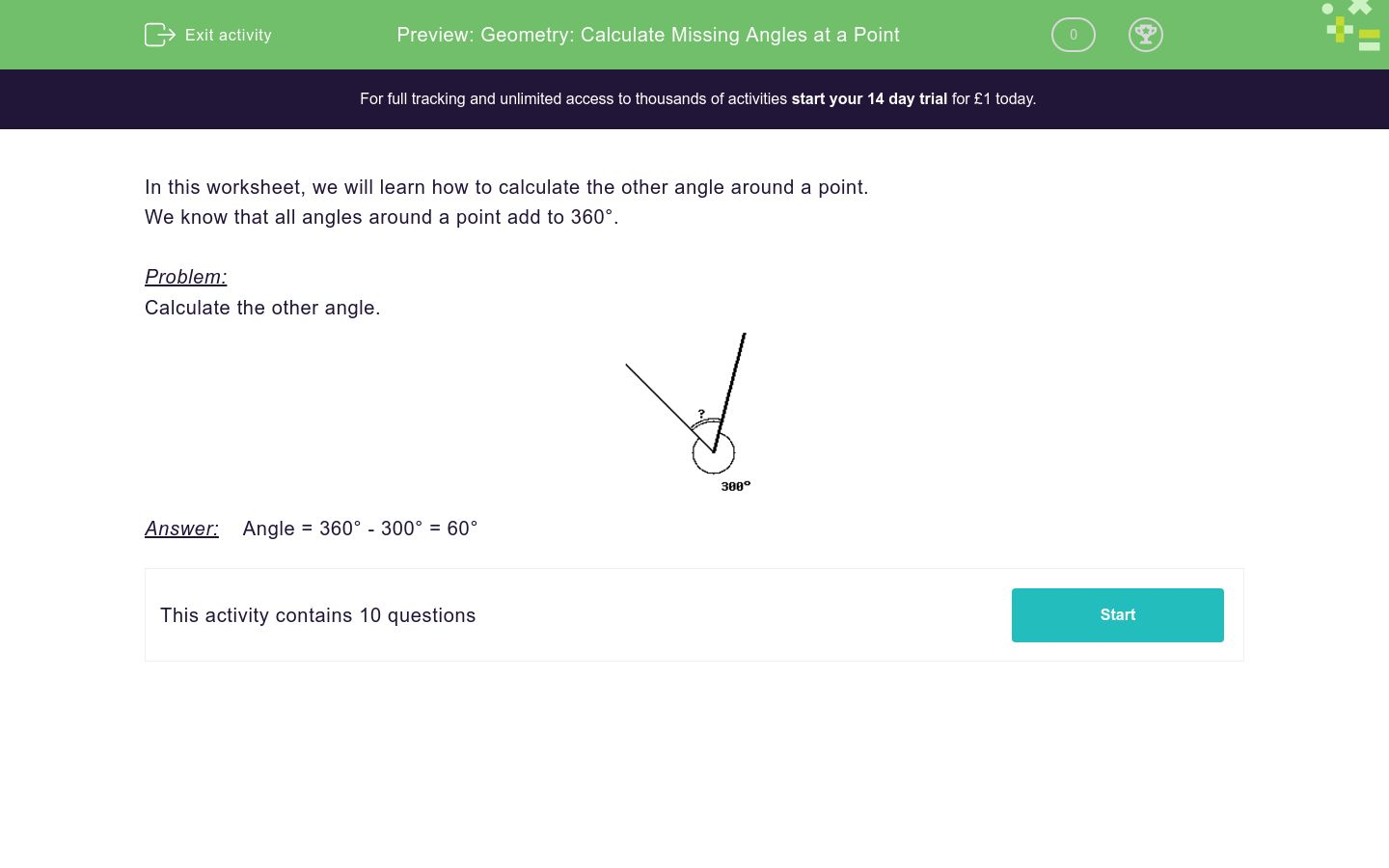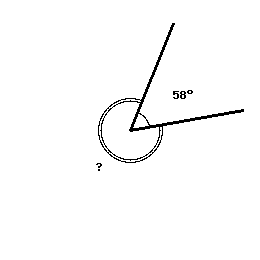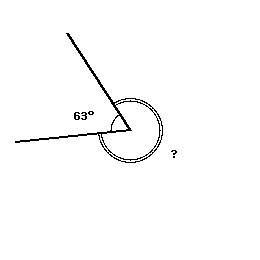# Geometry: Calculate Missing Angles at a Point

In this worksheet, students examine and calculate angles at the point where one whole turn is 360 degrees.Key stage:  KS 2

Curriculum topic:   Geometry: Properties of Shapes

Curriculum subtopic:   Identify Angles at a Point

Difficulty level:### QUESTION 1 of 10

In this worksheet, we will learn how to calculate the other angle around a point.

We know that all angles around a point add to 360°.

Problem:

Calculate the other angle.Answer:    Angle = 360° - 300° = 60°

Calculate the other angle.Calculate the other angle.Calculate the other angle.Calculate the other angle.Calculate the other angle.Calculate the other angle.Calculate the other angle.Calculate the other angle.Calculate the other angle.Calculate the other angle.• Question 1

Calculate the other angle.152
EDDIE SAYS
Remember, the angles around a point add up to 360.
So, 360 - 208 = 152
• Question 2

Calculate the other angle.302
EDDIE SAYS
360 - 58 = 302
• Question 3

Calculate the other angle.200
EDDIE SAYS
360 - 160 = 200
• Question 4

Calculate the other angle.313
EDDIE SAYS
360 - 47 = 313
• Question 5

Calculate the other angle.154
EDDIE SAYS
360 - 206 = 154
• Question 6

Calculate the other angle.285
EDDIE SAYS
360 - 75 = 285
• Question 7

Calculate the other angle.297
EDDIE SAYS
360 - 63 = 297
• Question 8

Calculate the other angle.60
EDDIE SAYS
360 - 300 = 60
• Question 9

Calculate the other angle.152
EDDIE SAYS
360 - 208 = 152
• Question 10

Calculate the other angle.313
EDDIE SAYS
360 - 47 = 313
---- OR ----

Sign up for a £1 trial so you can track and measure your child's progress on this activity.

### What is EdPlace?

We're your National Curriculum aligned online education content provider helping each child succeed in English, maths and science from year 1 to GCSE. With an EdPlace account you’ll be able to track and measure progress, helping each child achieve their best. We build confidence and attainment by personalising each child’s learning at a level that suits them.

Get started